# How to use DATEDIF Function in Excel

Try to type “DATEDIF” in a cell in your worksheet, you won’t find anything listed with this name.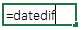Even if you check help system...

...there is nothing listed with this name.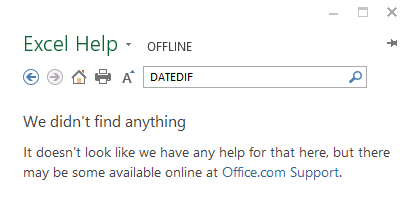But, if you step back to the help system of the Excel 2000, you will find DATEDIF function documented there.

Well, it’s a mystery that why Microsoft has hide the DATEDIF Function from functions list.

If you ask me, DATEDIF is the best function to get the difference between two dates.

And, one of its best examples is calculating a person’s age using his date of birth.

Now the question is:

If this function is not there, how we can use it in all the new versions of Excel?

We can use it like we use all the Excel functions. The only difference is when you try to insert it Excel will not show it’s syntax arguments.

So today, in this post, I’d like to share with you all the details about DATEDIF function.

Let’s get started.

## Syntax

DATEDIF(Date1,Date2,Interval)

Date1: The date from where you want to start the calculation of your interval. In simple words, it is the start date of the interval.

Date2: The date to which you want to calculate the interval. In simple words, it is the end date of your interval.

Interval: It represents the unit of the time, which will return from the function. We have six specific text strings to represent different time units.

## Using Different Intervals

You have 6 different types of intervals to calculate the difference between two dates.

### “y” for Years

It will return the complete years between the start date and the end date ignoring the months and days which are in excess.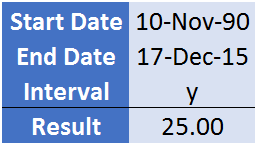In the above example, you have calculated the interval between two dates by using “y”.

If you actually check, the difference between these two dates is more than 25 years (37 days more).

But, it has only returned the completed years, that is 25.

### “m” for Months

It will return the number of complete months between the start date and end date by ignoring the excess days.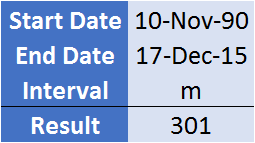It has returned 301 months in the result which are the total completed months ignoring excess 7 days.

### “d” for Days

It will return the total days between the start date and end date. As days are the smallest units between two dates, that’s why you will get total days here.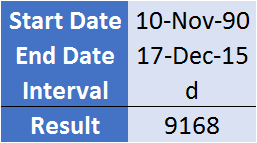In the above example, we have total days between the start and end date.

### “ym” for Months over Years

It will return numbers of the completed months over the completed years.

In simple words, if you have the total interval of 25 years 1 month and 7 days between two dates, it will return 1 month in the result.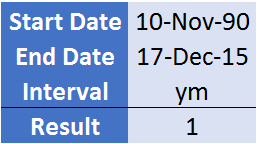In the above example, we have calculated the number of complete months left after competing years between the start date and end date.

### “yd” for Days over Years

It will return the number of the days over the complete years.

In the simple words, if you have a total interval of 25 years and 37 days between two dates, it will return 37 days in the result.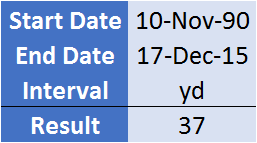In the above example, you have calculated the number of days left after the complete years between the start date and end date.

### “md” for Days over Months

It will return the excess number of days over the complete months.

In simple words, if you have the total interval of 301 months and 7 days between two dates, it will return 7 days in the result.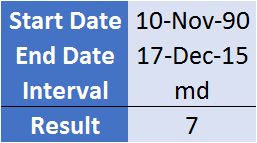In the above example, we have calculated the number of days left after the complete months between the start date and end date.

## Things To RememberPuneet is using Excel since his college days. He helped thousands of people to understand the power of the spreadsheets and learn Microsoft Excel. You can ﬁnd him online, tweeting about Excel, on a running track, or sometimes hiking up a mountain.

### 8 thoughts

1. =DATE(YEAR(F7),((INT((MONTH(F7)-1)/3)+1)*3)+1,1)-1
I need this formula to not future date. For example if i put in 08/2021 i need the result to be 08/2021 or if i put in 07/2021 to return it as 07/2021. I need for it to continue to calculate the last quarter for months before 07/20201.

2. Now I am learning Hidden Functions as well 🙂

Thanks Puneet..

3. Hi Puneet, is it possible to use this in Dax? Or indeed PowerQuery? Especially where one is required to sum up a list of start dates – end dates to get a subtotalled yy mm dd. I’ve been able to do this in excel but struggling with the other two…

4. Hi Puneet,

Would you be able to see working days only? i.e.: If a job card is 7 days old I would like to see how many work days (Monday – Friday) the device is in for repairs and not the entire period as we only count working days. Hope there is a way?

5. Really valuable tip. I’ve always done it using Int and Frac